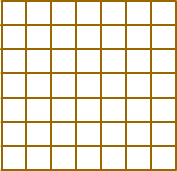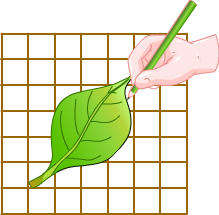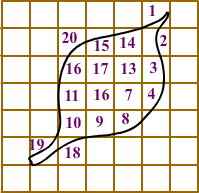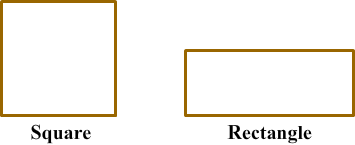# Applications of Area and Perimeter

Rate Lesson 0 stars
Common Core
• Lesson size:
Message preview:
Someone you know has shared lesson with you:

To play this lesson, click on the link below:

https://www.turtlediary.com/lesson/applications-of-area-and-perimeter.html

Hope you have a good experience with this site and recommend to your friends too.

Login to rate activities and track progress.
Login to rate activities and track progress.
CCSS.Math.Content.3.MD.D.8CCSS.Math.Content.4.MD.A.3
Perimeter

The perimeter is the distance around the outside of an object. The perimeter is found by adding the lengths of all of the sides of the object together.

For instance, perimeter of the given shape is calculated by adding together all the lengths of an object.The perimeter will be:

3 + 3 + 3 + 3 + 3 + 3 = 18

So, the perimeter is 18 cm.

Let's take a look at some examples:

Example 1

While making a card for your parents you want to put yarn around the card. Measure the sides to find out how much yarn is required.The length and the width of the card is 6 inches and 4 inches respectively.The perimeter of the card is:

6 + 4 + 4 + 6 = 20

So, 20 inches of yarn is required for the card.

Area

Area is the size of the surface of the object or the total amount of space that the object covers.Lets see how to find out the area of the given leaf.

To find out the area of such shapes we need to use grid paper.First we need to put the shape on a graph paper and draw the shape on it.Here we can notice that the squares don't match the shape exactly, therefore we can get an "approximate" answer.

Here we need to remember that,

• more than half a square counts as 1.
• less than half a square counts as 0.

Now count the number of squares:This is how we can calculate the area of an irregular shape.

To find the area of regular shapes such as square and rectangle can be calculated by the specific formula.

Area = Length x WidthLet us try out a question to find area and perimeter of shapes.

Example 1

Dalton's rectangular shaped field is 10 m long and 6 m wide. He wants to put a fence around the field to protect his field from animals. What is the length of wire required to complete the boundary of field ? He also wants to know how much area is required to be irrigated in his field. Can you help him?

Since, we are very familiar of the concept of area and perimeter, we can easily help him. To find the length of fence required to complete the boundary of field, we have to find the perimeter .

Add the lengths of all the sides of the rectangular field.

10 + 6 + 10 + 6 = 32

So, Dalton need 32 m of wire to put fence around the field.

Now, let us calculate the area of the field to find part to be irrigated.

Since, area of rectangle = Length x Width
So, area of part to be irrigated = 10 x 6
= 60 m2 .

Summary
• The perimeter is the distance around the outside of an object.
• The perimeter is found by adding the lengths of all of the sides of the object together.
• Area is the size of the surface of the object or the total amount of space that the object covers.
• Area of rectangle and square = Length x Width

## More Area and Perimeter Lessons

Become premium member to get unlimited access.